Differentiate cells that equals

In this article, we will learn about how to use Conditional formatting to Compare two arrays to show difference in excel.

Conditional Formatting Excel is used to highlight the data on the basis of some criteria. It would be difficult to see various trends just for examining your Excel worksheet. Conditional Formatting in excel provides a way to visualize data and make worksheets easier to understand.

How to solve the problem?

First we need to understand the logic behind this task. To compare list we need to match the cell values and keep a record of matched values. On the basis of the record we highlight the cells which differs from the other list.

First we will understand the logic behind it via using the formula on a data.

EXAMPLE:

Here we have a list of Names, One is name list and another is their respective country they belong to.

There are two methods to do the same but have a slight difference.

1. Case - sensitive formula
2. Not a case - sensitive formula

Case - sensitive formula

We will construct a formula to compare and highlight cells that match the value.

We need to highlight the country who are from the brasil. For this we will use EXACT function. EXACT function compares and returns TRUE if matches or else FALSE.

Generic formula:

= EXACT ( cell1, "value")

cell1 : first value of the list
value : value to match with, can be given as cell reference or with quote ( " ) character.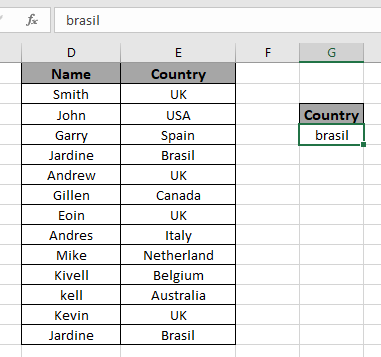To highlight those differences we will use the formula in conditional formatting  cell.
Now we use this formula to highlight cell values. For this we will use Conditional formatting tool of excel.
Now we will apply conditional formatting to the first list. Then we will proceed to the second list

Select the first array or array1.

Select Home > Conditional Formatting > New Rule.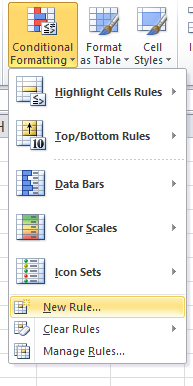A dialog box appears and choose Use a formula to determine which cells to format option. Then a formula box appears.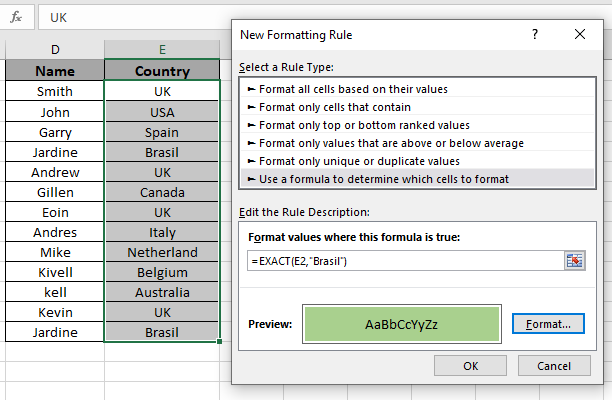Use the formula in the formula box.

= EXACT ( E2 , "Brasil" )

Explanation :

EXACT function matches the C2 cell value with "Brasil" and returns TRUE or FALSE.

Conditional formatting highlight the value for which the function returns TRUE.

Fill Format with Green colour and click OK.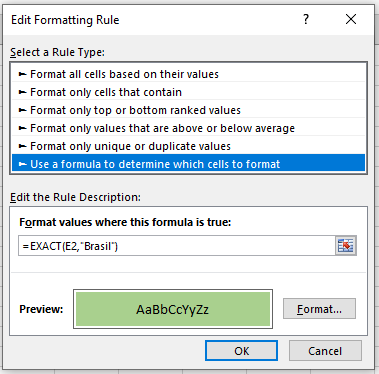Click Ok to get the names who belong from "Brasil".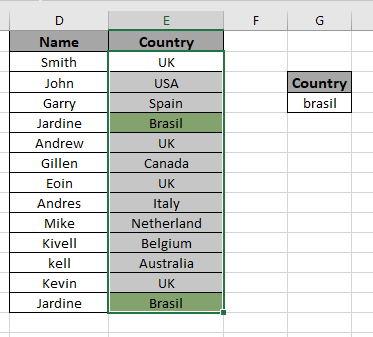As you can see excel change cell color based on value of another cell using Conditional formatting tool.

Not a case - sensitive formula

We will use a formula to compare and highlight cells that match the value.

We need to highlight the country who are from the UK. For this we will use cell reference option.

Syntax of generic formula:

= cell1 = fixed_cell_reference (value)

Variables:
cell1 : first value of the list
value : value to match with, can be given as cell reference or with quote ( " ) character.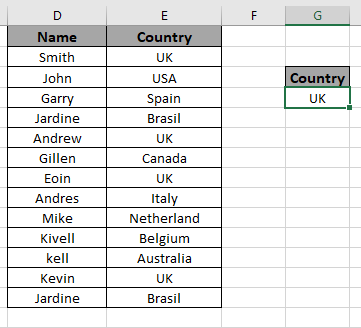To highlight those differences we will use the formula in conditional formatting  cell.

Now we use this formula to highlight cell values. For this we will use Conditional formatting tool of excel.

Now we will apply conditional formatting to the first list. Then we will proceed to the second list

Select the first array or array1.

Select Home > Conditional Formatting > New Rule.A dialog box appears and choose Use a formula to determine which cells to format option. Then a formula box appears.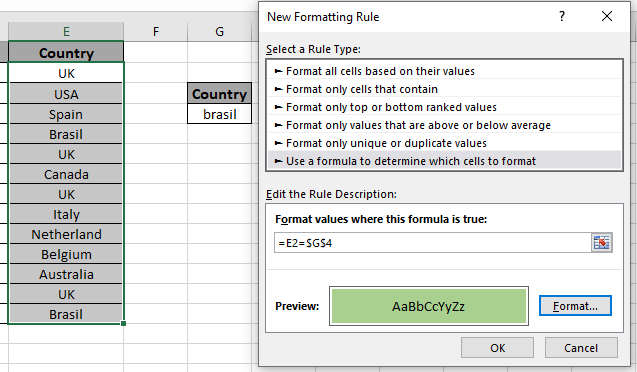Use the formula in the formula box.

= E2 = \$G\$4

Explanation :

The formula check the E2 cell value with the G4 cell value and returns TRUE or FALSE.

\$G\$4 : fixed cell reference format.

Fill Format with Green colour and click OK.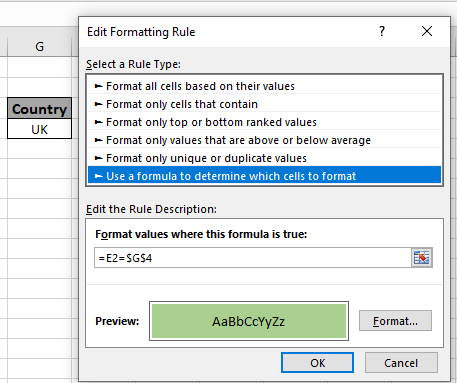Click Ok to get the names who belong from "Brasil".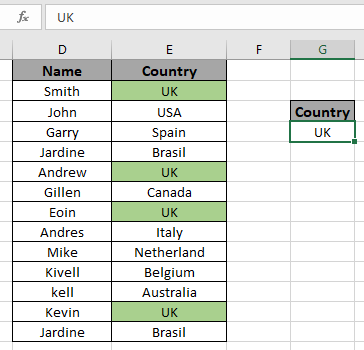As you can see excel change cell color based on value of another cell using Conditional formatting tool.

Notes:

1. Use the cell reference and fixed cell reference carefully.
2. Make sure to select the cell before using conditional formatting tool.
3. Text Value can be given as cell reference or directly in the function using quotes ( " ).
4. EXACT function is a case - sensitive function.

Hope you learned how to use conditional formatting in Excel used to Differentiate cells that equals and highlight the differences. Explore more conditional formulas in excel here. You can perform Conditional Formatting in Excel 2016, 2013 and 2010. If you have any unresolved query regarding this article, please do mention below. We will help you.

Related Articles:

How to use the Countif function in excel

Expanding References in Excel

Relative and Absolute Reference in Excel

Shortcut To Toggle Between Absolute and Relative References in Excel

Dynamic Worksheet Reference

All About Named Ranges In Excel

Total number of rows in range in excel

Dynamic Named Ranges in Excel

Popular Articles:

50 Excel Shortcut’s to Increase Your Productivity

The VLOOKUP Function in Excel

COUNTIF in Excel 2016

How to Use SUMIF Function in Excel

Terms and Conditions of use

The applications/code on this site are distributed as is and without warranties or liability. In no event shall the owner of the copyrights, or the authors of the applications/code be liable for any loss of profit, any problems or any damage resulting from the use or evaluation of the applications/code.